https://doi.org/10.5194/amt-13-4111-2020
https://doi.org/10.5194/amt-13-4111-2020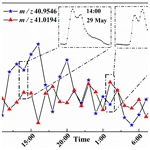# Development of an automatic linear calibration method for high-resolution single-particle mass spectrometry: improved chemical species identification for atmospheric aerosols

Shengqiang Zhu, Lei Li, Shurong Wang, Mei Li, Yaxi Liu, Xiaohui Lu, Hong Chen, Lin Wang, Jianmin Chen, Zhen Zhou, Xin Yang, and Xiaofei Wang
Abstract

The mass resolution of laser desorption ionization (LDI) single-particle aerosol mass spectrometry (SPAMS) is usually low (∼500), which has been greatly improved by the recent development of the delayed ion extraction technique. However, due to large fluctuations among LDI processes during each laser shot, accurate calibration of the mass-to-charge ratio for high-resolution SPAMS (HR-SPAMS) spectra is challenging. Here we developed an automatic linear calibration method to improve the accuracy of mass-to-charge (mz) measurement for single atmospheric aerosol particles. Laboratory-generated sea spray aerosol and atmospheric ambient aerosol were tested. After the calibration, the fluctuation ranges of the reference ions' (e.g., Pb+ and ${\mathrm{SO}}_{\mathrm{4}}^{+}$) mz reaches ±0.018 for sea spray aerosol and ±0.024 for ambient aerosol in average mass spectra. With such mz accuracy, the HR-SPAMS spectra of sea spray aerosol can easily identify elemental compositions of organic peaks, such as Cx, CxHy and CxHyOz. While the chemical compositions of ambient aerosols are more complicated, CxHy, CxHyOz and CNO peaks can also be identified based on their accurate mass. With the improved resolution, the time series of peaks with small mz differences can be separated and measured. In addition, it is also found that applying high-resolution data with enhanced mass calibration can significantly affect particle classification (identification) using the ART-2a algorithm, which classify particles based on similarities among single-particle mass spectra.

Share
Dates
1 Introduction

Atmospheric aerosols can significantly impact radiative forcing, cloud formation and human health (Ackerman et al., 2004; Zhang and Kin-Fai, 2012). They originate from various sources and undergo many atmospheric aging processes, resulting in an extremely complicated mixture of particles with a large range of sizes and chemical compositions. This mixture is usually referred to as the “mixing state”. Measurement of the aerosol mixing state requires single-particle characterization techniques. Utilizing laser ablation ionization of singe aerosol particles, single-particle aerosol mass spectrometry (SPAMS) has been widely used to measure chemical compositions, sizes and the refractory index of aerosols in real time (Moffet and Prather, 2009; Murphy, 2010; Sullivan and Prather, 2005). Based on this technique, ART-2a and other algorithms have been developed to classify the ambient particles based on their mass spectra and identify their sources (Reinard et al., 2007; Zelenyuk and Imre, 2009).

However, the SPAMS with laser desorption ionization (LDI) method has several serious limitations (Manuel et al., 2006; Wenzel et al.,  2003). A major issue is that the mass resolution of the SPAMS is relatively low (∼500) and the accuracy of mz (mass-to-charge ratio) is usually at the integer level, resulting in uncertainties about the identification of chemical species (Nash et al., 2006; Pratt and Prather, 2012; Qin et al., 2006). Due to the low mass resolution, many organic and inorganic peaks cannot be separated, such as ${\mathrm{K}}^{+}/{\mathrm{C}}_{\mathrm{3}}{\mathrm{H}}_{\mathrm{3}}^{+}$ from the mz peak of 39, Al+ ${\mathrm{C}}_{\mathrm{2}}{\mathrm{H}}_{\mathrm{3}}^{+}$ from the mz peak of 27 and ${\mathrm{CN}}^{-}/{\mathrm{C}}_{\mathrm{2}}{\mathrm{H}}_{\mathrm{2}}^{-}$ from the mz peak of −26 (Li et al., 2018). To better identify these particulate chemical species, a higher-mass-resolution version of SPAMS with better mz accuracy is needed.

Recently, Li et al. (2018) significantly increased SPAMS's mass resolution to ∼2000 by applying a delayed ion extraction technique, which combined a standard rectangular extraction pulse with an exponential pulse. This new SPAMS is called high-resolution SPAMS (HR-SPAMS). Unfortunately, in spite of resolution enhancement with this new technique, ion peak position is still very sensitive to initial ion coordinates and speed. Chudinov et al. (2019) have demonstrated that the ion peak shifts in 208Pb+ and ${}^{\mathrm{147}}\mathrm{Na}\left({\mathrm{NO}}_{\mathrm{3}}{\right)}_{\mathrm{2}}^{-}$ could be varied in the range of ±10 ns and the ion start position could be varied in the range of ±150µm. As a result, substantial peak jittering is observed when switching between mass spectra of each individual particle. This peak jittering leads to the fact that isotopic pattern identification by the averaged mass spectrum becomes more difficult (Chudinov et al., 2019). Furthermore, the peak jittering is different in each single-particle mass spectrum. In other words, the calibration parameter for each mass spectrum should be significantly different and calibration is required for each particle. Therefore, in order to obtain accurate mz, Chudinov et al. (2019) used several peaks with known mz to calibrate every SPAMS spectrum for Pb(NO3)2 and NaI particles produced from an atomizer.

In this study, we report a calibration method for single-particle high-resolution mass spectra data. Based on the assumption that the sea spray aerosol has relatively simple chemical composition, while the ambient aerosol has more complex chemical composition, the performance of the calibration method had been evaluated in detail for these two aerosol systems with different complexities. In addition, the impact of using high-resolution SPAMS data on particle classification by the ART-2a algorithm was assessed. An open-source code specific to HR-SPAMS was made, and we propose the principle of this calibration method can be applied to some similar instruments, such as a single-particle-mode aerosol mass spectrometer (AMS).

However, atmospheric particles are extremely complicated with a wide range of chemical compositions and sizes (Zhang et al., 2013), which brings much greater challenges to properly calibrating each SPAMS mass spectra and obtaining accurate mz measurement. We need to develop a new mass spectrometry (MS) calibration method for atmospheric aerosols and evaluate its performance comprehensively.

2 Experimental section

## 2.1 High-resolution single-particle aerosol mass spectrometry (HR-SPAMS)

The detailed description of the HR-SPAMS instrument (Hexin Analytical Instrument Co., Ltd., China) can be found elsewhere (Li et al., 2018). Briefly, an HR-SPAMS instrument consists of an aerodynamic lens as its particle inlet, a two-laser-beam system for particle sizing, a UV laser for LDI, and a bipolar time-of-flight (TOF) mass analyzer for the detection of positive and negative ions. Positive and negative ions are detected by two z-shaped bipolar TOF reflectron mass analyzers. The size detection range of the HR-SPAMS instrument is 200–2000 nm. As introduced before, this HR-SPAMS instrument uses a delayed ion extraction technique to enhance its mass resolution.

## 2.2 Laboratory-generated sea spray aerosol

Sea spray aerosol was produced by the water jet method. In a sea spray aerosol production tank, a seawater jet hit the seawater surface and produced bubbles, which would rise to the surface and burst. The bubble-bursting process produces sea spray aerosols. Seawater was collected at Fengxian, Shanghai (3092 N, 12147 E) on 30 March 2019 (Fig. S1 in the Supplement).

## 2.3 Ambient aerosol sampling

Ambient aerosol sampling was conducted at Fudan University, Shanghai (3120 N, 12130 E) on 29 May 2019 (Fig. S1). The ambient particles were dried by a diffusional dryer before being sampled by the HR-SPAMS instrument.

3 Development of calibration methods

## 3.1 Automatic linear calibration method

To improve the accuracy of mz for HR-SPAMS spectra, an automatic linear calibration method has been developed. Noticeably, due to the technical limitation of data acquisition, the whole HR-SPAMS spectrum is not continuous but divided by a large number of mz bins, which are described in Fig. S2 (a partial enlarged detail in the single-particle mass spectra) and can be viewed as the probability density histogram of mz. Here we denote “mz bin value” as the median mz value of each bin.

The linear calibration method is described as the following steps:

Step 0. The SPAMS data were coarsely calibrated by the traditional method, which usually selected a few particles with distinct ion patterns; i.e., the molecular composition of some distinct peaks in the mass spectra can be easily identified. Then, the time of flight of these peaks and the true mz of the corresponding ions were used to calculate a set of calibration parameters for positive and negative spectra. The parameters were finally applied to the whole mass spectra dataset, and coarse calibration was completed.

Step 1. A pool of ion peaks in the single-particle mass spectra was selected as the potential mz calibration reference ions. The selection criteria are (1) these peaks should be present in most of the spectra and (2) the identification of these ion peaks should not be significantly affected by other adjacent peaks. For example, 27Al+ was not selected, as its adjacent peak 27C2H3 may affect the peak shape and identification of 27Al.

According to previous research, possible peak assignments for the mz of reference ions for sea spray aerosol and ambient aerosol are listed in Table 1 (Bertram et al., 2018; Collins et al., 2014; Tsunogai et al., 1972; Wang et al., 2016, 2019). For sea spray aerosol, according to several studies (Bertram et al., 2018; Collins et al., 2014; Tsunogai et al., 1972), the reference ions with mz values of 23, 24, 39, −35 and −37 were 23Na+, 24Mg+, 39K+, ${}^{-\mathrm{35}}{\mathrm{Cl}}^{-}$ and ${}^{-\mathrm{37}}{\mathrm{Cl}}^{-}$, respectively. And Collins et al. (2014) show that the reference ions with mz values of 81, 83, −26, −42, −58, −129 and −131 were 81Na2Cl, 83Na2Cl, ${}^{-\mathrm{26}}{\mathrm{CN}}^{-}$, ${}^{-\mathrm{42}}{\mathrm{CNO}}^{-}$, ${}^{-\mathrm{58}}{\mathrm{NaCl}}^{-}$, ${}^{-\mathrm{129}}{\mathrm{MgCl}}_{\mathrm{3}}^{-}$ and ${}^{-\mathrm{131}}{\mathrm{MgCl}}_{\mathrm{3}}^{-}$, respectively (Collins et al., 2014). Due to the fact that Na, Mg and K were abundant in sea spray aerosol, the reference ions with mz values of 113 and 115 should be 113K2Cl+ and 115K2Cl+. Thus, in this study, we select 23Na+, 24Mg+, 39K+, 81Na2Cl+, 83Na2Cl+, 113K2Cl+, 115K2Cl+, ${}^{-\mathrm{35}}{\mathrm{Cl}}^{-}$, ${}^{-\mathrm{37}}{\mathrm{Cl}}^{-}$, ${}^{-\mathrm{26}}{\mathrm{CN}}^{-}$, ${}^{-\mathrm{42}}{\mathrm{CNO}}^{-}$, ${}^{-\mathrm{129}}{\mathrm{MgCl}}_{\mathrm{3}}^{-}$, ${}^{-\mathrm{131}}{\mathrm{MgCl}}_{\mathrm{3}}^{-}$ and ${}^{-\mathrm{58}}{\mathrm{NaCl}}^{-}$ as the potential reference ions for sea spray aerosols.

Table 1Possible peak assignments for the mz of reference ions for sea spray aerosol and ambient aerosol.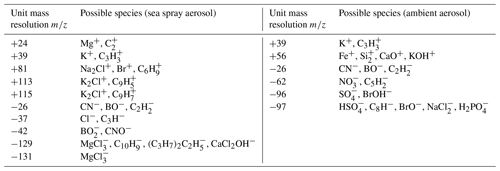For the ambient aerosol, according to the previous ambient SPAMS measurements (Wang et al., 2016, 2019), the reference ions with mz values of 12, 23, 36, 39, 56, 207, 208 and 209 were assigned to 12C+, 23Na+, ${}^{\mathrm{36}}{\mathrm{C}}_{\mathrm{3}}^{+}$, 39K+, 56Fe+, 207Pb+, 208Pb+ and 209Pb+ and the reference ions with mz values of −26, −35, −46, −62, −96 and −97 were assigned to ${}^{-\mathrm{26}}{\mathrm{CN}}^{-}$, ${}^{-\mathrm{35}}{\mathrm{Cl}}^{-}$, ${}^{-\mathrm{46}}{\mathrm{CNO}}^{-}$, ${}^{-\mathrm{62}}{\mathrm{NO}}_{\mathrm{2}}^{-}$, ${}^{-\mathrm{96}}{\mathrm{SO}}_{\mathrm{4}}^{-}$ and ${}^{-\mathrm{97}}{\mathrm{HSO}}_{\mathrm{4}}^{-}$, respectively. So in this study, we select 12C+, 23Na+, 39K+, ${}^{\mathrm{36}}{\mathrm{C}}_{\mathrm{3}}^{+}$, 56Fe+, 208Pb+, 206Pb+, 207Pb+, ${}^{-\mathrm{62}}{\mathrm{NO}}_{\mathrm{3}}^{-}$, ${}^{-\mathrm{26}}{\mathrm{CN}}^{-}$, ${}^{-\mathrm{35}}{\mathrm{Cl}}^{-}$, ${}^{-\mathrm{96}}{\mathrm{SO}}_{\mathrm{4}}^{-}$, ${}^{-\mathrm{46}}{\mathrm{NO}}_{\mathrm{2}}^{-}$ and ${}^{-\mathrm{97}}{\mathrm{HSO}}_{\mathrm{4}}^{-}$ as the potential reference ions for ambient aerosols.

Step 2. A set of reference ions was chosen from the potential reference ion pool for each spectrum. The selection was based on the absolute ion intensity of the reference ions in each spectrum. They must be greater than a threshold; e.g., we set 15 arbitrary units (a.u.) for ambient aerosol and 8 a.u. for sea spray aerosol, respectively. A particle was discarded from the spectra database if it did not have enough reference ions (the minimum number of reference ions was set to be five) in either the positive or negative mass spectrum.

Step 3. The reference ions were used to calibrate mz for mass spectra of each particle. As introduced before, an HR-SPAMS spectrum consists of a number of bins. The measured mz bin values of the reference ions mentioned in Step 0 were calibrated based on their theoretic (or true) mz bin values. A linear regression between the two sets of variables (measured vs. theoretic mz bin values) was conducted, and two calibration parameters (a slope and an intersect) could be obtained. Then we used these parameters to make the calibration for every bin value in these mass spectra. Finally, the mz of the whole spectrum was corrected. Thus, we assigned an mz bin value to each corrected mz based on the proximity principle. Finally, mass spectra with well-calibrated bin values can be obtained for each single particle.

A GUI program for this automatic linear calibration method was developed for the sake of easy use (Fig. 1). The MATLAB codes for this GUI and the automatic linear calibration method are open access and available at https://data.mendeley.com/datasets/zhx56bzjfj/1 (last access: 29 July 2020).

## 3.2 Evaluation of the calibration method

In this study, a total of 5130 sea spray aerosol particles and 5007 ambient aerosol particles were analyzed. And 4624 sea spray particles and 1409 ambient particles were successfully calibrated. Some fraction of particles was filtered because the mass spectrum of these particles did not have five or more reference peaks to conduct the calibrations. To mitigate this problem, we propose some adjustments in the next section. Figure 2 shows the calibration curves for a randomly selected sea spray aerosol particle and ambient aerosol particle. The adjusted R2 coefficients of both calibration curves are equal to ∼1, demonstrating that this calibration method is effective and accurate. All the slopes and intercepts of the linear calibration can be found in Figs. S3 and S4. In addition, Fig. 3a and b report a comparison of mz distributions of reference ions between, before and after automatic linear calibration. The results show that the fluctuations in the reference ion mz values were significantly reduced after automatic linear calibration. The average mz deviation of the reference ions was reduced from ∼0.04 to ∼0.001 for sea spray aerosol and from ∼0.035 to ∼0.006 for ambient aerosol, respectively.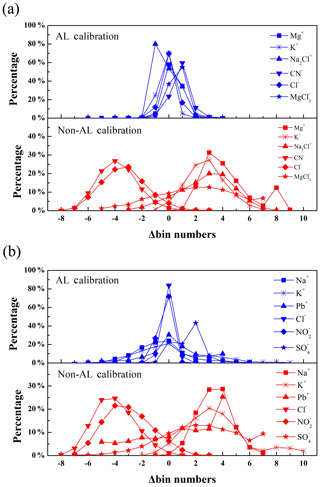Figure 3Probability distributions of the marker peak locations before and after automatic linear calibration for (a) sea spray aerosol and (b) ambient aerosol.

## 3.3 Automatic linear calibration method with a larger reference ion pool

It is important to note that a large number of ambient particles were filtered because their spectra did not have five or more reference peaks to conduct calibrations. Specifically, only ∼ 29.0 % of total ambient particles had a sufficient number of reference ions in their positive spectra. To solve this problem, extra reference ions, including 67VO+, ${}^{\mathrm{67}}{\mathrm{C}}_{\mathrm{5}}{\mathrm{H}}_{\mathrm{7}}^{+}$, ${}^{\mathrm{89}}{\mathrm{C}}_{\mathrm{7}}{\mathrm{H}}_{\mathrm{5}}^{+}$, ${}^{\mathrm{89}}{\mathrm{Na}}_{\mathrm{2}}{\mathrm{BO}}_{\mathrm{2}}^{+}$, ${}^{\mathrm{102}}{\mathrm{C}}_{\mathrm{8}}{\mathrm{H}}_{\mathrm{6}}^{+}$ and ${}^{\mathrm{102}}{\mathrm{CaNO}}_{\mathrm{3}}^{+}$, were added into the original positive reference ion pool. Obviously, these ions share the same integer mz value with other ions. We needed to identify them using additional information other than their integer mz values.

The specific reference ions were determined by their coarsely calibrated mz. Table S2 in the Supplement shows that the mz deviation ranges of the reference positive ambient ions in coarsely calibrated spectra before automatic linear calibration were around 0.011–0.048, while the mz differences between 67VO+ and ${}^{\mathrm{67}}{\mathrm{C}}_{\mathrm{5}}{\mathrm{H}}_{\mathrm{7}}^{+}$, ${}^{\mathrm{89}}{\mathrm{C}}_{\mathrm{7}}{\mathrm{H}}_{\mathrm{5}}^{+}$ and ${}^{\mathrm{89}}{\mathrm{Na}}_{\mathrm{2}}{\mathrm{BO}}_{\mathrm{2}}^{+}$, and ${}^{\mathrm{102}}{\mathrm{C}}_{\mathrm{8}}{\mathrm{H}}_{\mathrm{6}}^{+}$ and ${}^{\mathrm{102}}{\mathrm{CaNO}}_{\mathrm{3}}^{+}$ were 0.1213, 0.0622 and 0.083, respectively, which were larger than the mz deviations of these reference ions in coarsely calibrated spectra. Therefore, the coarsely calibrated spectra can be used to determine these specific reference ions. With these additional potential reference ions, a total of 2490 ambient particles were calibrated, much more than in the previous analysis (1409 ambient particles). The deviations from the theoretical mz for applying this expanded ion pool are summarized in Table S3. The average mz deviation of the reference ions is ∼0.0068.

4 Application to atmospheric aerosol measurement

## 4.1 HR-SPAMS measurement of sea spray aerosol

SPAMS data usually contain a large number of individual mass spectra. It is impossible to manually analyze every spectrum from a large dataset. Averaging a number of mass spectra is often preferred. However, to obtain an averaged high-resolution spectrum, each spectrum must be well calibrated. Therefore, it would be very interesting to see what new information can be obtained from HR-SPAMS measurement of aerosols with the automatic calibration method. Figure 4 reports the average positive and negative mass spectra for the laboratory-generated sea spray aerosols, and the error limits mean the concluded accepted error range. Similar to the low-resolution sea spray aerosol mass spectra, they contain major peaks of Na+, Mg+, K+, Na2Cl+, CN, Cl, CNO, NaCl, ${\mathrm{NaCl}}_{\mathrm{2}}^{-}$ and ${\mathrm{MgCl}}_{\mathrm{3}}^{-}$, as well as many smaller peaks, such as peaks of Ca+, ${\mathrm{SiO}}_{\mathrm{2}}^{-}$ and ${\mathrm{SiO}}_{\mathrm{3}}^{-}$, and ${\mathrm{KCl}}_{\mathrm{2}}^{-}$. With the improved mz measurement, many peaks, which cannot be determined by integer resolution mass spectra, can now be clearly identified (Table 2). For example, the ion with an mz of 27.0267 is ${\mathrm{C}}_{\mathrm{2}}{\mathrm{H}}_{\mathrm{3}}^{+}$ rather than Al+. The ion with an mz of 76.9336 is CaCl+ rather than ${\mathrm{C}}_{\mathrm{6}}{\mathrm{H}}_{\mathrm{5}}^{+}$. And some sulfur-containing organic ions, such as CS+, can be determined. Surprisingly, we can identify the presence of ${\mathrm{HCO}}_{\mathrm{2}}^{-}$ and ${\mathrm{CaCO}}_{\mathrm{3}}^{-}$, demonstrating that carbohydrates are contained in sea spray aerosols.

Table 2Peak identification of important chemical species in sea spray aerosols. The first column is the measured mz for peaks. The second and third columns show the theoretical mz bin value and theoretical mz value of most possible species for each peak.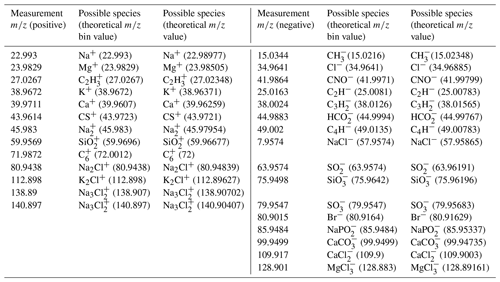## 4.2 HR-SPAMS measurement of atmospheric aerosol

Laboratory-generated sea spray aerosol can be viewed as a relatively simple aerosol system, while the chemical compositions of ambient aerosols are much more complicated. Figure 5 shows the averaged HR-SPAMS mass spectra of the ambient aerosols sampled at Fudan University, Jiangwan Campus, on 29 May 2019. With the improved mz measurement, many organic ions, such as Cx, CxHy and CxHyOz, can be directly identified (Table 3). Also, we can separate the organic and inorganic species more directly with the high mass resolution. For instance, ${\mathrm{C}}_{\mathrm{6}}{\mathrm{H}}_{\mathrm{8}}^{+}$ can be clearly distinguished from possible interference of ${\mathrm{Ca}}_{\mathrm{2}}^{-}$, ${\mathrm{TiO}}_{\mathrm{2}}^{+}$ and NaKO+, and C10H can also be identified instead of a possible assignment as ${\mathrm{NaSO}}_{\mathrm{4}}^{-}$. More importantly, 139C2H3O5S (with the theoretical mz value of −138.97) can be clearly distinguished from other possible assignments, such as ${}^{\mathrm{139}}{\mathrm{C}}_{\mathrm{11}}{\mathrm{H}}_{\mathrm{7}}^{-}$ with the mz value of −139.55 and ${}^{\mathrm{139}}{\mathrm{AsO}}_{\mathrm{4}}^{-}$ with the mz value of −138.90. Moreover, 153C3H5O5S with the theoretical mz value of −152.986 can be distinguished from other possible assignments, such as ${}^{\mathrm{153}}{\mathrm{C}}_{\mathrm{12}}{\mathrm{H}}_{\mathrm{9}}^{-}$ with the mz value of −153.070 and ${}^{\mathrm{153}}{\mathrm{Na}}_{\mathrm{2}}{\mathrm{Cl}}_{\mathrm{3}}^{-}$ with the mz value of −152.883. These two important organic ion peaks have been suggested to be the characteristic ion peaks for the organosulfates in secondary organic aerosols (Surratt et al., 2010, 2007).

Table 3Peak identification of important chemical species in ambient aerosols. The first column is the measured mz for each peak. The second and third columns show the theoretical mz bin value and theoretical mz value of most possible species for each peak.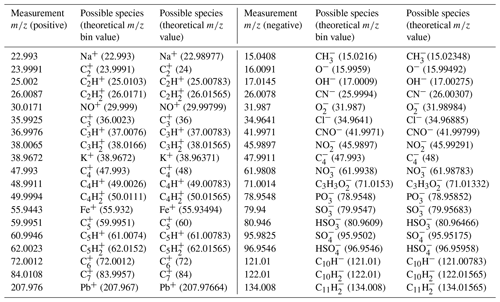## 4.3 Time variation in HR-SPAMS measurement

With the high mass resolution of HR-SPAMS and enhanced mz calibration, we were able to obtain an average mass spectrum from many particles. The accurate mz values in the average mass spectrum can be used to separate peaks with close mz values and track their intensity variations. Here we conducted a time variation measurement for ambient aerosol from 11:00 LT (UTC+8) on 29 May to 11:00 LT on 30 May 2019. We selected the first 500 particles collected by SPAMS during every hour for elemental analysis. Figure 6 shows that the peak at mz 41 has a bimodal structure, whose mz values were 40.9546±0.0105 and 41.0194±0.0105, respectively. Thus the peak with the smaller mz is an isotope of K+ (theoretical mz value of ${}^{\mathrm{41}}{\mathrm{K}}^{+}=\mathrm{40.96182}$, theoretical mz bin value of ${}^{\mathrm{41}}{\mathrm{K}}^{+}=\mathrm{40.9667}$; this peak also follows the isotopic pattern of K) and the other peak should be ${\mathrm{C}}_{\mathrm{3}}{\mathrm{H}}_{\mathrm{5}}^{+}$ (theoretical mz value 41.03913, theoretical mz bin value 41.03). Figure 6 shows that HR-SPAMS was able to separately measure the time series of these two peaks with a small mz difference. In contrast, it is impossible for low-resolution SPAMS (LR-SPAMS) to provide such detailed time variation measurement of these peaks.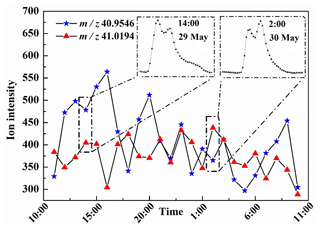Figure 6Time series of peak intensities at mz 40.95 and mz 41.01. Time is local time.

## 4.4 Particle classification by ART-2a

The adaptive resonance theory neural network (ART-2a) is a widely used method to classify particles based on the similarity among their mass spectra (Song et al., 1999). Here we make a comparison of ART-2a classification between the HR-SPAMS data and traditional low-resolution SPAMS (LR-SPAMS) data. Particles with the positive and negative spectra were analyzed by ART-2a with a learning rate of 0.05, a vigilance factor of 0.7 and an iteration number of 20. The previous ambient aerosol SPAMS dataset (1400 particles) used for the matrix size of the ART-2a is around 7×107. The LR-SPAMS data, whose mz was at the integer level, were generated by summing high-resolution SPAMS peaks in each integer mz bin. The classification results show that the HR-SPAMS data were grouped into 93 categories and the top 45 categories accounted for 96 % of all particles. The particle numbers of the first eight categories were 122, 101, 99, 86, 82, 70, 68 and 60, respectively. In contrast, the LR-SPAMS data were only grouped into 33 categories in total and the top 20 categories accounted for 96 % of all particles. The particle numbers of the first eight categories were 170, 118, 107, 107, 106, 92, 90 and 88, respectively. The detailed results can be found in Figs. S5–S6. Obviously, ART-2a classification of high-resolution SPAMS data generated more particle categories. This is mainly because HR-SPAMS mass spectra can differentiate peaks with close mz values, which may be viewed as one peak in LR-SPAMS data.

The ART-2a classification of the HR-SPAMS results (Fig. S6) shows that the signal at 23Na+ in Type 2HR was stronger than in Type 1HR while the signals at 26CN and 42CNO were weaker in Type 1HR. Meanwhile the averaged mass spectra of the Type 2HR showed the presence of 206Pb+, 207Pb+ and 208Pb+, which are known to be harmful to human health (Das et al., 2018; Peng et al., 2020). Furthermore, particles of Type 2HR contained abundant secondary inorganic components like ${\mathrm{NO}}_{\mathrm{2}}^{-}$, ${\mathrm{NO}}_{\mathrm{3}}^{-}$ and ${\mathrm{SO}}_{\mathrm{4}}^{-}$, which originated from the aerosol aging processes (Dall'Osto and Harrison, 2012; Ma et al., 2016). In contrast, these first two particle types were lumped together into Type 1LR in the LR-SPAMS classification results (Fig. S5). Due to the merge of these two particle types, Pb+ ions were not significant. Meanwhile, types 3HR, 4HR and 5HR classification results contain strong signals at 26CN, 42CNO, ${}^{\mathrm{46}}{\mathrm{NO}}_{\mathrm{2}}^{-}$, ${}^{\mathrm{62}}{\mathrm{NO}}_{\mathrm{3}}^{-}$ and ${}^{\mathrm{97}}{\mathrm{HSO}}_{\mathrm{4}}^{-}$, suggesting that these three types were from biomass burning or residential cooking burning. K+ is also another feature of this type of particle emission (Bi et al., 2011; Hudson et al., 2004). There were obvious relative ion intensity differences at 26CN, 42CNO, ${}^{\mathrm{46}}{\mathrm{NO}}_{\mathrm{2}}^{-}$ and ${}^{\mathrm{62}}{\mathrm{NO}}_{\mathrm{3}}^{-}$ among these types, which implied that these three particle types might be from different burning sources or experienced different levels of aging (Luo et al., 2020). While these three particle types were lumped together as Type 2LR, this critical information which could be potentially used to distinguish particle sources and aging processes was lost. Additionally, Type 7HR can be assigned as organic and elemental carbon (OCEC) type, based on its strong signals at ${\mathrm{C}}_{x}^{+}$, ${\mathrm{C}}_{x}{\mathrm{H}}_{y}^{+}$ and ${\mathrm{C}}_{x}{\mathrm{H}}_{y}{\mathrm{O}}_{z}^{+}$. Particles of this type may come from the primary emission sources, and the emitted black carbon particles would also form particles of this type after absorbing some low-volatile organic compounds in the atmosphere (Sodeman et al., 2005). ${}^{\mathrm{97}}{\mathrm{HSO}}_{\mathrm{4}}^{-}$ can be observed to have a weaker signal than ${}^{\mathrm{62}}{\mathrm{NO}}_{\mathrm{3}}^{-}$ and ${}^{\mathrm{46}}{\mathrm{NO}}_{\mathrm{2}}^{-}$, which implied that the secondary reaction of SO2 as the precursor of ${\mathrm{HSO}}_{\mathrm{4}}^{-}$ was not significant in the particle surface for ECOC-type particles in this study (Sullivan and Prather, 2007). In contrast, the classification results of the LR-SPAMS were not so clear and generated fewer particle types. Given HR-SPAMS spectra have much more detailed chemical information about particles, we would propose that the ART-2a classification of HR-SPAMS might be more accurate.

5 Conclusion

An automatic linear calibration method has been developed for data analysis of high-resolution SPAMS data. This technique can significantly improve the mz accuracy of SPAMS spectra for atmospheric aerosol samples. The analysis of HR-SPAMS data for laboratory-generated sea spray aerosols shows many details of their chemical composition. For example, many organic ions, such as ${\mathrm{C}}_{\mathrm{2}}{\mathrm{H}}_{\mathrm{3}}^{+}$ and CS+, can be directly determined. The chemical compositions of ambient aerosols are much more complicated. It is found that, besides major ions (e.g., Na+, K+, Ca+, Fe+, Cl, CN, ${\mathrm{NO}}_{\mathrm{3}}^{-}$ and ${\mathrm{HSO}}_{\mathrm{4}}^{-}$), CxHy, CxHyOz and CNO can be identified. With this method, HR-SPAMS can also determine the time series of organic and inorganic peaks, whose mz values are very close to each other (e.g., 41K+ with the theoretical mz value of 40.96182 and ${\mathrm{C}}_{\mathrm{3}}{\mathrm{H}}_{\mathrm{5}}^{+}$ with the theoretical mz value of 41.03913). Important organic ion peaks, such as tracer peaks for secondary organic matter like 139C2H3O5S and 153C3H5O5S, can be identified. More importantly, our ART-2a classification from the HR-SPAMS dataset clearly showed a particle type containing heavy metals like Pb+, which was obviously ignored in the ART-2a classification from the LR-SPAMS dataset. More particle types were generated by the ART-2a classification of HR-SPAMS data compared to that of LR-SPAMS data, as the original biomass burning particle type can be divided into three more detailed types based on the different signals of 26CN, 42CNO and ${}^{\mathrm{46}}{\mathrm{NO}}_{\mathrm{2}}^{-}$ and of other organic species like CxHy and CxHyOz, implying different aerosol aging processes or burning conditions. Such detailed information may be critical to studying the aging processes and source appointment of atmospheric aerosols. There is a deficiency in this HR-SPAMS calibration method, which has shown that some fraction of particles cannot be calibrated due to the presence of weak signals of the marker ions. It can be mitigated by applying some additional marker ions. All the automatic linear calibration method codes specific for HR-SPAMS are open access and can be found at https://github.com/zhuxiaoqiang-fdu/zhuxiaoqiang-fdu. And we proposed the principle of this calibration method can be adopted in other aerosol mass spectrometers.

Code and data availability

All the raw data and automatic linear calibration method codes specific for HR-SPAMS are open access and can be found at https://doi.org/10.17632/zhx56bzjfj.1 (Zhu, 2020).

Supplement

Author contributions

XY and XW supervised this study. XW and SZ designed the calibration and data analysis methods. SW and SZ performed the sea spray aerosol and ambient aerosol experiment. SZ wrote the open-source code for calibration and data analysis of the single-particle mass spectra and made the GUI program with suggestions from XW and XY. XW and SZ prepared the manuscript with contributions from all co-authors.

Competing interests

The authors declare that they have no conflict of interest.

Acknowledgements

This work was partially supported by the National Natural Science Foundation of China and Shanghai Natural Science Foundation. The authors thank Hexin Analytical Instrument Co., Ltd., China, for providing HR-SPAMS.

Financial support

This research has been supported by the National Natural Science Foundation of China (grant nos. 41827804, 41775150, 21906024 and 91544224) and the Shanghai Natural Science Foundation (grant no. 19ZR1404000).

Review statement

This paper was edited by Pinhua Xie and reviewed by three anonymous referees.

References

Ackerman, A. S., Kirkpatrick, M. P., Stevens, D. E., and Toon, O. B.: The impact of humidity above stratiform clouds on indirect aerosol climate forcing, Nature, 432, 1014–1017, 2004.

Bertram, T. H., Cochran, R. E., Grassian, V. H., and Stone, E. A.: Sea spray aerosol chemical composition: elemental and molecular mimics for laboratory studies of heterogeneous and multiphase reactions, Chem. Soc. Rev., 47, 2374–2400, https://doi.org/10.1039/C7CS00008A, 2018.

Bi, X., Zhang, G., Li, L., Wang, X., Li, M., Sheng, G., Fu, J., and Zhou, Z.: Mixing state of biomass burning particles by single particle aerosol mass spectrometer in the urban area of PRD, China, Atmos. Environ., 45, 3447–3453, 2011.

Chudinov, A., Li, L., Zhou, Z., Huang, Z., Gao, W., Yu, J., Nikiforov, S., Pikhtelev, A., Bukharina, A., and Kozlovskiy, V.: Improvement of peaks identification and dynamic range for bi-polar Single Particle Mass Spectrometer, Int. J. Mass Spectrom., 436, 7–17, 2019.

Collins, D. B., Zhao, D. F., Ruppel, M. J., Laskina, O., Grandquist, J. R., Modini, R. L., Stokes, M. D., Russell, L. M., Bertram, T. H., Grassian, V. H., Deane, G. B., and Prather, K. A.: Direct aerosol chemical composition measurements to evaluate the physicochemical differences between controlled sea spray aerosol generation schemes, Atmos. Meas. Tech., 7, 3667–3683, https://doi.org/10.5194/amt-7-3667-2014, 2014.

Dall'Osto, M. and Harrison, R. M.: Urban organic aerosols measured by single particle mass spectrometry in the megacity of London, Atmos. Chem. Phys., 12, 4127–4142, https://doi.org/10.5194/acp-12-4127-2012, 2012.

Das, R., Bin Mohamed Mohtar, A. T., Rakshit, D., Shome, D., and Wang, X.: Sources of atmospheric lead (Pb) in and around an Indian megacity, Atmos. Environ., 193, 57–65, 2018.

Hudson, P. K., Murphy, D. M., Cziczo, D. J., Thomson, D. S., Gouw, J. A. D., Warneke, C., Holloway, J., Jost, H. J., and Hübler, G.: Biomass-burning particle measurements: Characteristic composition and chemical processing, J. Geophys. Res., 109, D23S27, https://doi.org/10.1029/2003JD004398, 2004.

Li, L., Liu, L., Xu, L., Li, M., Li, X., Gao, W., Huang, Z., and Cheng, P.: Improvement in the Mass Resolution of Single Particle Mass Spectrometry Using Delayed Ion Extraction, J. Am. Soc. Mass Spectr., 29, 2105–2109, 2018.

Luo, J., Zhang, J., Huang, X., Liu, Q., Luo, B., Zhang, W., Rao, Z., and Yu, Y.: Characteristics, evolution, and regional differences of biomass burning particles in the Sichuan Basin, China, J. Environ. Sci., 89, 35–46, 2020.

Ma, L., Li, M., Huang, Z., Li, L., Gao, W., Nian, H., Zou, L., Fu, Z., Gao, J., Chai, F., and Zhou, Z.: Real time analysis of lead-containing atmospheric particles in Beijing during springtime by single particle aerosol mass spectrometry, Chemosphere, 154, 454–462, 2016.

Manuel, D. O., Harrison, R. M., Beddows, D. C. S., Freney, E. J., Heal, M. R., and Donovan, R. J.: Single-particle detection efficiencies of aerosol time-of-flight mass spectrometry during the North Atlantic marine boundary layer experiment, Environ. Sci. Technol., 40, 5029–5035, 2006.

Moffet, R. C. and Prather, K. A.: In-situ measurements of the mixing state and optical properties of soot with implications for radiative forcing estimates, P. Natl. Acad. Sci. USA, 106, 11872–11877, 2009.

Murphy, D. M.: The design of single particle laser mass spectrometers, Mass Spectrom. Rev., 26, 150–165, 2010.

Nash, D. G., Baer, T., and Johnston, M. V.: Aerosol mass spectrometry: An introductory review, Int. J. Mass Spectrom., 258, 2–12, 2006.

Peng, M., Zhao, C., Ma, H., Yang, Z., Yang, K., Liu, F., Li, K., Yang, Z., Tang, S., Guo, F., Liu, X., and Cheng, H.: Heavy metal and Pb isotopic compositions of soil and maize from a major agricultural area in Northeast China: Contamination assessment and source apportionment, J. Geochem. Explor., 208, 106403, https://doi.org/10.1016/j.gexplo.2019.106403, 2020.

Pratt, K. A. and Prather, K. A.: Mass spectrometry of atmospheric aerosols-Recent developments and applications. Part II: On-line mass spectrometry techniques, Mass Spectrom. Rev., 31, 17–48, 2012.

Qin, X., Bhave, P. V., and Prather, K. A.: Comparison of Two Methods for Obtaining Quantitative Mass Concentrations from Aerosol Time-of-Flight Mass Spectrometry Measurements, Anal. Chem., 78, 6169–6178, 2006.

Reinard, M. S., Adou, K., Martini, J. M., and Johnston, M. V.: Source characterization and identification by real-time single particle mass spectrometry, Atmos. Environ., 41, 9397–9409, 2007.

Sodeman, D. A., Toner, S. M., and Prather, K. A.: Determination of Single Particle Mass Spectral Signatures fromLight-Duty Vehicle Emissions, Environ. Sci. Technol., 39, 4569–4580, 2005.

Song, X.-H., Hopke, P. K., Fergenson, D. P., and Prather, K. A.: Classification of Single Particles Analyzed by ATOFMS Using an Artificial Neural Network, ART-2A, Anal. Chem., 71, 860–865, 1999.

Sullivan, R. C. and Prather, K. A.: Recent advances in our understanding of atmospheric chemistry and climate made possible by on-line aerosol analysis instrumentation, Anal. Chem., 77, 3861–3885, 2005.

Sullivan, R. C. and Prather, K. A.: Investigations of the Diurnal Cycle and Mixing State of Oxalic Acid in Individual Particles in Asian Aerosol Outflow, Environ. Sci. Technol., 41, 8062–8069, 2007.

Surratt, J. D., Kroll, J. H., Kleindienst, T. E., Edney, E. O., Claeys, M., Sorooshian, A., Ng, N. L., Offenberg, J. H., Lewandowski, M., and Jaoui, M.: Evidence for organosulfates in secondary organic aerosol, Environ. Sci. Technol., 41, 517–527, 2007.

Surratt, J. D., Chan, A. W. H., Eddingsaas, N. C., Chan, M. N., Loza, C. L., Kwan, A. J., Hersey, S. P., Flagan, R. C., Wennberg, P. O., and Seinfeld, J. H.: Reactive intermediates revealed in secondary organic aerosol formation from isoprene, P. Natl. Acad. Sci. USA, 107, 6640–6645, 2010.

Tsunogai, S., Saito, O., Yamada, K., and Nakaya, S.: Chemical composition of oceanic aerosol, J. Geophys. Res., 77, 5283–5292, 1972.

Wang, H., An, J., Shen, L., Zhu, B., Xia, L., Duan, Q., and Zou, J.: Mixing state of ambient aerosols in Nanjing city by single particle mass spectrometry, Atmos. Environ., 132, 123–132, 2016.

Wang, H., Shen, L., Yin, Y., Chen, K., Chen, J., and Wang, Y.: Characteristics and mixing state of aerosol at the summit of Mount Tai (1534 m) in Central East China: First measurements with SPAMS, Atmos. Environ., 213, 273–284, 2019.

Wenzel, R. J., Liu, D. Y., Edgerton, E. S., and Prather, K. A.: Aerosol time-of-flight mass spectrometry during the Atlanta Supersite Experiment: 2. Scaling procedures, J. Geophys. Res., 108, 8427, https://doi.org/10.1029/2001JD001563, 2003.

Zelenyuk, A. and Imre, D.: Beyond single particle mass spectrometry: multidimensional characterisation of individual aerosol particles, Int. Rev. Phys. Chem., 28, 309–358, 2009.

Zhang, G., Bi, X., Chan, L. Y., Wang, X., Sheng, G., and Fu, J.: Size-segregated chemical characteristics of aerosol during haze in an urban area of the Pearl River Delta region, China, Urban Climate, 4, 74–84, 2013.

Zhang, R. J. and Kin-Fai, H. O.: The Role of Aerosol in Climate Change,the Environment, and Human Health, Atmospheric and Oceanic Science Letters, 5, 156–161, 2012.

Zhu, S.: Al-CAL-SPAMS, Mendeley Data, v1, https://doi.org/10.17632/zhx56bzjfj.1, 2020.